# Plus Two Physics Chapter Wise Questions and Answers Chapter 13 Nuclei

Students can Download Chapter 13 Nuclei Questions and Answers, Plus Two Physics Chapter Wise Questions and Answers helps you to revise the complete Kerala State Syllabus and score more marks in your examinations.

## Kerala Plus Two Physics Chapter Wise Questions and Answers Chapter 13 Nuclei

### Plus Two Physics Nuclei NCERT Text Book Questions and Answers

Question 1.
Obtain the binding energy of a nitrogen nucleus ($$_{ 7 }^{ 14 }{ N }$$) Given m $$_{ 7 }^{ 14 }{ N }$$ = 14.00307 u.
Here Z = 7 and A = 14, A – Z = 14 – 7 = 7
∴ Mass defect
= [Z mH + (A – Z)mn – Mn] u
= (7 × 1.00783 + 7 × 1.00867 – 14.00307) u
= (7.05481 + 7.06069-14.00307) u = 0.11243 u
Since I u = 931.5 MeV
∴ B.E. of 14N = 0.11243 × 931 Mev
= 104.7 MeV.Question 2.
Obtain approximately the ratio of the nuclear radii of the gold isotope $$_{ 79 }^{ 197 }$$Au and the silver isotope $$_{ 47 }^{ 107 }$$Ag
Here A1 = 197 and A2 = 107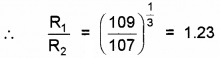Question 3.
How long an electric lamp of 100 W can be kept glowing by fusion of 2.0 kg of deuterium? The fusion reaction as.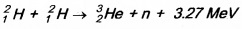Number of deuterium atoms is 2 kg
= $$\frac{6.023 \times 10^{23}}{2}$$ × 2000 = 6.023 × 1026
Energy released when 6.023 × 1023 nuclei of deuterium fuse together= 15.42 × 1013J = 15.42Ws
Power of lamp = 100 W
If the lamp glows fortime t, then electric energy consumed = 100 t
∴ 100 t = 15.42 × 1033
∴ t = 0.1542 × 1013s
$$=\frac{0.1542 \times 10^{13}}{365 \times 86400} y$$
or t = 4.0 × 104y.Question 4.
From the relation R = R0 A$$\frac{1}{3}$$, Where R0 is a constant and A is the mass number of a nucleus show that the nuclear matter density is nearly constant (i.e. Independent of A).
Density of nucleus(p) It is defined as the nuclear mass per unit volume.Thus the nuclear density is of the order of 1017kgm-3 and is independent of its mass number. Therefore, all nuclei have the same approximate density.

### Plus Two Physics Nuclei One Mark Questions and Answers

Question 1.
Fusion reaction takes place at high temperature because
(a) nuclei break up at high temperature
(b) atoms get ionized at high temperature
(c) kinetic energy is high enough to overcome the coulomb repulsion between nuclei
(d) molecules break up at high temperature
(c) kinetic energy is high enough to overcome the coulomb repulsion between nuclei.Question 2.
Give the relation between half-life and mean life
t1/2 = 0.693τ.

Question 3.
Write down the expression for nuclear radius
R = R0A1/3.

Question 4.
Complete the given nuclear reaction### Plus Two Physics Nuclei Two Mark Questions and Answers

Question 1.
A beam of radio active radiation is unaffected in a combined electric and magnetic field in mutually perpendicular direction. A boy argues that, it is essentially a gamma ray. Do you agree with him. Justify your answer.
It need not be gama ray. It can be α or β ray as the electric and magnetic field are in mutually perpendicular direction.Question 2.
Pick the odd one out of the following.
a.

1. Curie
2. Roentgen
3. Becquerel
4. Rutherford

b.

1. γ – decay
2. β – decay
3. β+ – decay
4. α – decay

a. odd one:
2. Roentgen.

b. odd one:
1. γ – decay

Question 3.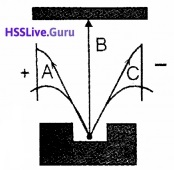1. Identify the radiation B. Give reason.
2. If the electric field is replaced by magnetic field perpendicular and into the plane of the paper, identify the particle deflecting towards right (C).

1. Gamma-ray. It has no change
2. C which is β rayQuestion 4.
Two radioactive substances P and Q have half-life 6 months and 3 months respectively. Find the ratio of the activity of these two materials after one year.
Activity R = λN
R1 = λ1N1
R2 = λ2N2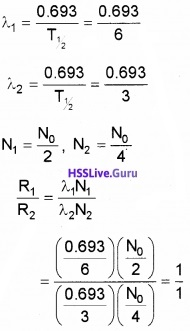R1: R2 = 1: 1Question 5.
Match the following.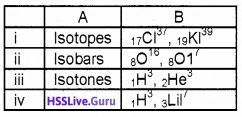Question 6.
Suppose you are a health physicist and you are being consulted about a spill occurred in a radio chemistry lab. The isotope spilled out in the lab was 500 micro cure of 131Ba which has half-life of 12 – days.

1. What is the decay constant of 131Ba
2. Your recommendation is to clear the lab until the radiation level is down to 1 micro curie. How length will the lab have to be closed.

1.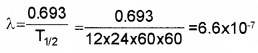2. 9 × 12 = 108 days.Question 7.
Classify the following statement in to nuclear fission and nuclear fusion

2. Can be controlled
3. Reaction is spontaneous
4. Can’t proceed as a chain reaction

1. fission
2. fission
3. fission
4. fusion

Question 8.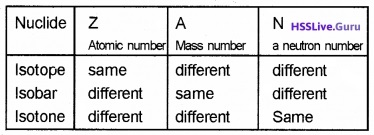### Plus Two Physics Nuclei Three Mark Questions and Answers

Question 1.
1. Nuclear fusion can liberate more energy than nuclear fission. But nuclear fusion is not commonly used in energy production. Why?
2. Match the following.

 A B 1. Nuclear fission β – decay 2. Nuclear fusion Hydrogen spectrum 3. Transition between atomic energy level Nuclei with low atomic numbers 4. Electron emission from nucleus Generally possible for nuclei with high atomic number Photoelectric emission

1. Nuclear fusion is a thermonuclear reaction. It occurs at high temperature (106k) and it is difficult to attain such a high temperature. Hence fusion is not commonly used in energy production.

2.

 A B 1. Nuclear fission Generally possible for nuclei with high atomic number 2. Nuclear fusion Nuclei with low atomic numbers 3. Transition between atomic energy level Hydrogen spectrum 4. Electron emission from nucleus β – decayQuestion 2.
β – Particles does not exist inside a nucleus. But it is emitted from the nucleus!

1. What is β Particle
2. What happens to nucleus of a atom, when a particle is emitted? Explain
3. Why β particle is emitted from the nucleus?

1. It is electron
2. When α is emitted, mass number decreases to 4 and atomic decreases by 2.
3. To get stability, β is emitted from the nucleus.

Question 3.
Nuclear radius depends on the mass number of the element.

1. Write down the expression for nuclear radius.
2. Prove that the density of the nucleus is independent of mass number A.

1. R = R0 A1/3.

2. Density of nucleus = (mass of the nucleus)/ (volume of the nucleus)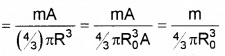This shows that nuclear density is independent of mass number.Question 4.
Match the following.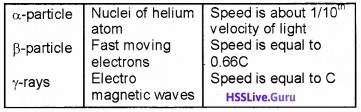Question 5.
The fission of one nucleus of 92U239 release 200 Mev of energy.

1. What is meant by fission
2. Express 200 Mev energy in joule
3. How many fission of 92U239 should occur per send for producing a power of 1 Mev

1. The splitting of heavy nucleus into two nucleus is called fission.

2. E = 200Mev
= 200 × 106ev
E = 200 × 106 × 1.6 × 10-19J
= 3.2 × 10-11J.

3. Number of fission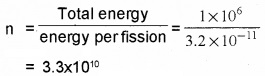Question 6.
Match the following.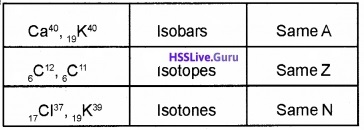Question 7.
Size of the nucleus increases with number of nucleons. As size increases, volume increase (ie mass number increases).

1. Based on the above facts find an expression for radius of nucleus.
2. Calculate radius of 13Al27 nucleus. The constant R0 = 1.2 fermi.

1. R = R0A1/3
2. R = 1.2 × (27)1/3 fermi = 3.6 fermi.Question 8.
Binding energy curve shows the variation of Binding energy per nucleon of nuclei with mass number.
1. Binding energy per nucleon is maximum for mass number……. (1)
2. The figure shows dis integration of Deuteron. (2)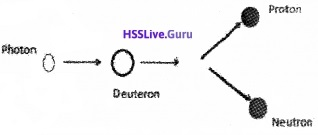What should be the frequency of the incident photon to break Deuteron into proton and neutron?
Mass of proton mp = 1,007276u.
Mass of neutron mn = 1,008665u
Mass of deuteron = 2.013553u
1. 56

2. Mass defect = (1.007276 + 1.008665) – 2.013553 = 0.002388u
Binding Energy = 0.002388 × 931 MeV = 2.223
MeV Energy supplied to the photon
= 2.223 × 106 × 1.6 × 10-19
= 3.56 × 10-13J
Frequency of photon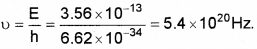Question 9.
The figure shows the potential energy of a pair of nuclear particles and their distance of separation in Fermi (fm).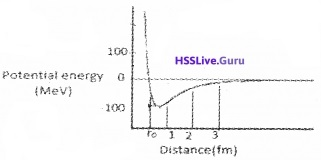1. Fill in the blanks.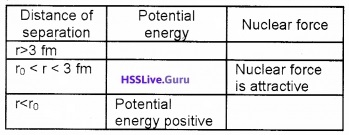2. What conclusion do you obtain about the nature of nuclear force from the graph.
1.2. The nuclear force is a short range force.

### Plus Two Physics Nuclei Four Mark Questions and Answers

Question 1.
Atomic mass of $$_{ 8 }^{ 16 }{ O }$$ is found to be 16.0000u

1. what is the mass of $$_{ 8 }^{ 16 }{ O }$$ nucleus
(Hint: mass of an electron = 0.00055u)
2. determine the total mass of the constituents particles of the $$_{ 8 }^{ 16 }{ O }$$ nuclei.
(Hint : Mass of neutron = 1.00864 u, Mass of proton = 1.007274 u)
3. Give a general expression for mass defect and explain what is binding energy?
4. Binding energy per nucleon is lower for both very light nuclei (Z ≤ 10) and very heavy nuclei (Z ≥ 70) Justify a nuclear fission and fusion

1. Mass of nucleus = (8 × 1.00864 + 8 × 1.007274) amu.

2. (8 × 1.00864 + 8 × 1.007214 + 8 × 0.0055)amu

3. ∆m = [ZMp + (A-Z) Mn – M)
The energy equivalent to mass defect is called binding energy.

4. When two light nuclei are combined to form a heavy nucleus, the binding per nucleon increases. Hence the stability of atom increases. When heavy nucleus split into two light nuclei; B.E. for nucleon also increases.Question 2.
Classify the following into alpha, beta, and gama

1. Similar to fast moving electron
2. It is an electromagnetic wave
3. Similar to helium nucleus
4. Travel with 1/10th the velocity of light
5. Travel with 99/100th the velocity of light
6. Travel with the velocity of light
7. Positively charged
8. Negatively charged

1. beta particle – 1, 3, 8
2. Gama ray – 2, 6
3. alpha particle – 3, 4, 7Question 3.
a. Two protons and two neutrons may bound to form a single particle it is called

1. α particle
2. β particle
3. deuteron
4. triton

b. If such a particle is emitted what changes will occur in the nucleus
c. The penetrating power of the particle is very small in air. Why?
d. If the particle is projected upward in a uniform magnetic field with direction perpendicular to plane in ward, towards which plate (A or B) it is deflected? To find this which law is applied?a.
1. α particle.

b. Mass number decreases to 4 and atomic number decreases to 2

c. penetrating power of the particle is very small in air:
(i) Mass of α particle is 4. Hence penetrating power of the particle is small.

d. Towards A (left) Flemings left hand rule is used.Question 4.
Nuclear radius depends on the mass number of the element.

1. Write down the expression for nuclear radius. (1)
2. Prove that the density of the nucleus is independent of mass number A. (2)
3. Read the following statement and choose the correct option.

“Electric dipole moment is zero for nuclei in stationary state.” (1)
Assertion:
All nuclei have spherical symmetry about the centre of mass.
Reason:
The zero dipole moment for stationary nuclei is due to the symmetry about the centre of mass.

• Assertion and reason are true.
• Assertion is false reason is true
• Assertion is true reason is false
• Assertion and reason are false.

1. R = R0A1/3.

2. Density of nucleus = (mass of the nucleus)/(volume of the nucleus)This shows that nuclear density is independent of mass number.

3.
(ii) Assertion is false reason is true.

Question 5.1. Which process is represented by the equation?
2. What happens to the parent nucleus after the process?
3. After this process the nucleus will be in a higher energy state. How will it come to ground state?
4. If a proton splits in a nucleus what are the changes. Represent it with an equation.

1. β – emission.
2. Atomic increases to one unit. But mass number remains constant
3. The parent atom comes to ground state by emitting gamma ray.
4. P → n + e+ + ν
Atomic number decreases by one unit. But mass number remains constant.

### Plus Two Physics Nuclei Five Mark Questions and Answers

Question 1.
Rutherford and Soddy’s laws of radioactivity explain the rate of decay of radioactive material.

1. Arrive at the expression for the number of radio active atoms of a radioactive material remaining after an interval of time. (2)
2. Draw the curve showing the variation of log$$\left(\frac{N}{N_{0}}\right)$$ with time. (1)
3. Two radioactive substances P and Q have half life 6 months and 3 months respectively. Find the ratio of the activity of these two materials after one year. (2)

1. According to Law of Radioactive decay,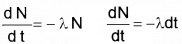Integrating
InN = -λt + C ………(1)
C is the constant of integration. To get value of C, let us assume that initially (t=0) the number of nuclei be N0.
∴ C = In N0
Substituting for C in equation (1) we get,
In N – In N0 = -λt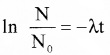$$\frac{\mathrm{N}}{\mathrm{N}_{0}}$$ e-λt
N = N0e-λt2.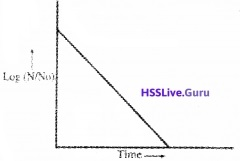3. Activity R = λN
R1 = λ1N1
R2 = λ2N2Question 2.
a. In the given figure a radioactive source is placed inside a lead block. Identify the rays incident on the photographic plates.b. Which of the following statement is correct.

1. Gamma rays consist of high energy neutrons.
2. Alpha rays are equivalent to singly ionized He atoms.
3. Protons and neutrons have exactly the same mass.
4. Beta rays are same as cathode rays.

c. How many alpha and beta particles are emitted in the following reaction.a.• 1 – Alpha
• 2-Gamma
• 3 – Beta

b.
4. Beta rays are same as cathode rays

c.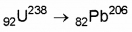Difference in mass number = 32
Mass number of one alpha particle = 4
Hence number of alpha particles = 8
Change in atomic number = 10
Change in atomic number due to alpha particles = 16
Charge of beta particles = -1
Hence number of beta particles emitted = 6Question 3.
The figure shows a nuclear reactor based on thermal neutron fission.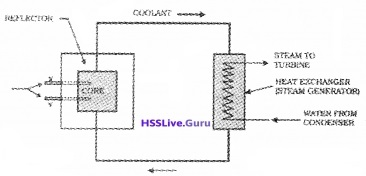1. The energy of thermal neutrons is……..ev. (1)
2. Name the parts labelled X and Y in the figure. (1)
3. Write the function of X and Y (1)
4. The multiplication factor has great significance in nuclear reactor. Give reason. (2)